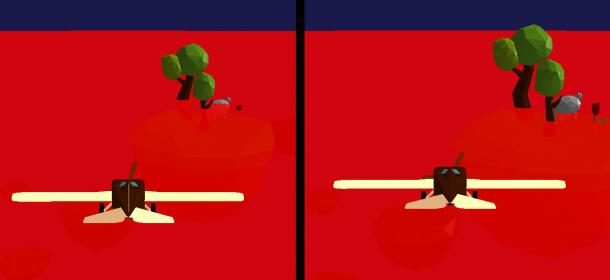# Plane intersection on the fragment shader

Hi everyone!

I would like to be able to do raycast cast test against a plane in the fragment shader, in order to do post process FX. Howver if the Ray-Plane intersection seems to works, the plane appears to move up or down depending on the elevation of the camera.

Here is the vertex shader, I draw a rectangle at the corner of the screen and send the current pixel in world space to the fragment buffer:

``````
uniform mat4 InverseViewProjectionMatrix;

out vec4 pixel;

void main(void)
{
// Draw a rectangle at the corner of the screen
vec2 vertices =
vec2(vec2(-1.0,  1.0), vec2(-1.0, -1.0), vec2( 1.0, -1.0),
vec2( 1.0, -1.0), vec2( 1.0,  1.0), vec2(-1.0,  1.0));

gl_Position = vec4(vertices[gl_VertexID], 1.0, 1.0);

// Get the projected current pixel in world space (it will be the
// 'end' of the ray)
vec4 P = InverseViewProjectionMatrix * vec4(vertices[gl_VertexID], 1.0, 1.0);
P = P / P.w;

pixel = P;

}
``````

And here is the fragment shader where, for each pixels, I raycast the plane and draw a red fragment if it intersect. I also send the DepthBuffer, previously computed, in order to not draw the plane on an object that would be upfront.

``````uniform mat4 InverseViewProjectionMatrix;
uniform vec3  CameraPosition;

in vec4 pixel;

uniform sampler2D DepthMapTexture;

out vec4 color;

void
main(void)
{
vec3 F = vec3(0, 1, 0);   // Plane normal
vec3 C = CameraPosition;  // Start of the ray
vec3 P = vec3(pixel);     // 'End' of the ray
vec3 ray = normalize(P - C);

float pn = dot(C, F);
float nd = dot(ray, F);

// Initialize the color to 0 (transparent)
color = vec4(0.0);

// make sure the dot product is not equal to zero
// Check `nd &lt; 0.0f` for just one side of the plane
if (abs(nd) > 0.0001f) {

// Project the current fragment in the worldspace in order to get the
// depth value.
vec4 wspace = gl_FragCoord;
wspace.x /= 1920.0; // TODO use screen value from an uniform
wspace.y /= 1080.0;
wspace.z /= wspace.w;

float depth = texture(DepthMapTexture, vec2(wspace)).r;

// Convert the value from the depth buffer to a linear depth. This will
// allow to check if a fragment of a mesh is before the fog or inside it.
float near = 0.1;
float far = 1000.0;
float z = depth;
float linearDepth = (2.0 * near * far) / (far + near - z * (far - near));

// -5.0 is the distance of the plane on the normal (0, 1, 0)
// We hit (and set a transparent red color) if `t &gt;= 0.0` (plane
// intersection) and `t &lt; linearDepth` (no object in front of).
float t = (-5.0 - pn) / nd;
if (t >= 0.000 && t < linearDepth) {
color = vec4(1, 0, 0, 0.8);
}
}
}
``````

Here is the result:Plane descent On the left the plane is at an altitude of 1.0 (above the plane) then I descent a bit and we can see on the right that the plane has also moved down and we can perceive now the top of the flower. While the plane should have not moved.

Any idea why it does that?

Thanks

This transforms NDC Z (which ranges from -1 to +1) to eye-space Z, with Z[sub]eye[/sub]=near when Z[sub]ndc[/sub]=-1 and Z[sub]eye[/sub]=far when Z[sub]ndc[/sub]=1, so [var]near[/var] and [var]far[/var] need to be negative with the usual conventions. But if [var]z[/var] is a depth value read from a texture, it’s probably going to be between 0 and 1, so you need e.g. z=(depth*2)-1 to convert depth to NDC Z.

Hi GClements,

Thanks for your answer! Indeed I’ve spent hours trying to understand why my ray wasn’t correct while the issue where somewhere else.
It now works with `z=(depth*2)-1`.

Fulezi.